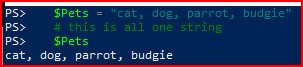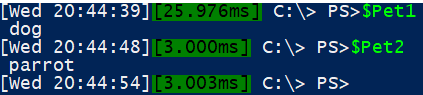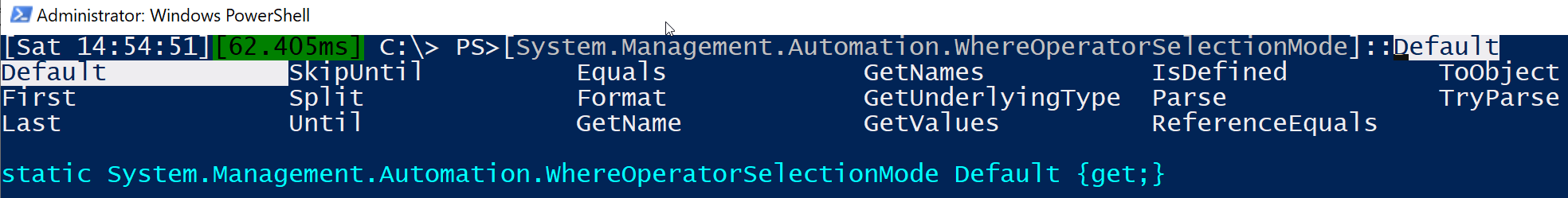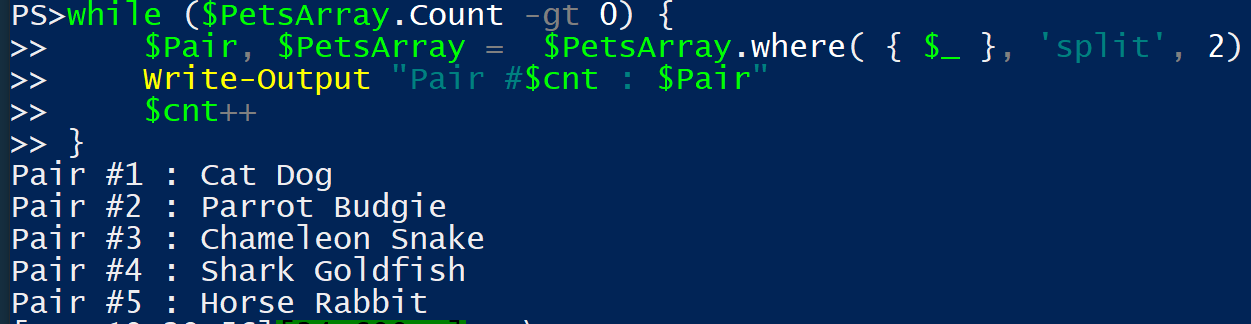Perhaps one of the most common PowerShell commandlets is Where-Object which lets you filter items in the pipeline, preventing unwanted items from the left operator being passed to the right operator. There are many commadlets that have a similar (in name and functionality) .Net method and in our case this is the .where method. Now the sacrifice we have to make when using .Net methods in PowerShell is that they dont play all that well with the PowerShell pipeline so you need to weigh the benefits of each and decide thoughtfully which to use. Here I will make a case for using the .Net Where method for a specific functionality.

First, some background understanding we will take a look at strings and delimiters and assignment of values.

Let’s create a list of pets

``````\$Pets = "cat, dog, parrot, budgie"
# this is all one string
\$Pets
``````Now, we can split the string using the -split operator and then we are given output that is similar to an array. This means we can get specific values from the array by specifying the element index (zero-based) number that we are interested in.

``````# we can split on a given character - note in the output the character gets consumed in the splitting
\$pets -split ','

# this output is an array so we can ask for specific elements by their ID
(\$pets -split ',') # specify a single ID
(\$pets -split ',')[0..2] # specify a range of element IDs
(\$pets -split ',')[1, 2] # specify individual element IDs
``````

This last option where we specify two or more elements is interesting. We can actually use that in a variable assignment line like this.

``````\$Pet1, \$Pet2 = (\$pets -split ',')[1, 2]

\$Pet1
\$Pet2
``````OK then, that’s pretty neat, assigning two variables with values from a result that gives two values. You can do this with more but that is perhaps the topic of another blog one day.

Keep this process in mind while we take a look at the .Net string method where.

``````# putting that to one side, let's take a look at .Where method
# Lets create an array to work with
\$PetsArray = (\$pets -split ',')
# filtering with the PowerShell native where-object commandlet
\$PetsArray | where-object {\$_ -like "*a*"}

# similar filter with the .Net where method
\$PetsArray.Where({\$_ -like "*a*"})
``````

Well, the output is the same, the syntax isn’t very different, just what differences are there? One down-side, as already mentioned, the .Net method doesnt work in a PowerShell pipeline but on the positive side, it is often faster when dealing with big arrays. I tested this a little and it is approx 50% faster on a test array of 1M items. Of course test this yourself, my testing might not represent your use case.

but what you dont often encounter are the extras …

### What extras?!

``````# we can go and look at online references or run this line to see the options in the error message!
\$PetsArray.Where()
# "Cannot find an overload for ".Where({ expression } [, mode [, numberToReturn]])" "
``````

### What does that tell us?

{expression} shown inside curly braces is a mandatory paramter. That makes sense this is the fundamental logic of the where operator that we want to have filtering our data.

[mode] a parameter in square brackets means it is optional

[numbertoreturn] square brackets so also optional

Now we know {expression} - That is what we have used and we can safely assume that numbertoreturn is an integer. So we need to find the acceptable values for mode and to do that we can get the command line to show us by typing `[System.Management.Automation.WhereOperatorSelectionMode]::` and then pressing Ctrl+Space

``````# we have to explore the WhereOperatorSelectionMode enumerator
[System.Management.Automation.WhereOperatorSelectionMode]::
``````From the above screen capture we can see a variety values that we can investigate:

• First
• Last
• SkipUntil
• Split
• Until
Example Description Output
`\$PetsArray.where({\$_ -like "*a*"})` The default use-case that filters items based on the filter specification Cat, Parrot
`\$PetsArray.where({\$_ -like "*a*"}, 'First')` Returns the first item encountered by the filter specification and no more. Cat
`\$PetsArray.where({\$_ -like "*a*"}, 'Last')` Returns the last item encountered by the filter specification and no more. Parrot
`\$PetsArray.where({\$_ -like "*g*"}, 'SkipUntil')` Once a value matches the filter specification, return all items thereafter. Dog, Parrot, Budgie
`\$PetsArray.where({\$_ -like "*g*"}, 'Split')` returns a set of items based on the filter specification and a set containing items that dont match Dog, Budgie, Cat, Parrot
`\$PetsArray.where({\$_ -like "*r*"}, 'Until)` Return everything until an item matches the filter specification cat, dog

Some pretty useful options to know about right there but let’s focus on the split option, combining it with the trick we saw up above where we can set two variables to output values in one line.

This example below shows us setting two variables (`\$sql` and `\$NotSQL`) to the output of Get-Service passed through a .where filter that also uses the split option.

``````\$sql, \$NotSQL = (Get-Service).where({\$_.name -like "*sql*"},'split')
``````

If we review the values of `\$sql` and `\$NotSQL` we will see that `\$sql` contains all of the services on the local computer that have their name contain the string ‘sql’ and `\$NotSQL` will have every other service. Now this isnt the only way to achieve this, you can use Group-Object to get a similar effect but its always good to have options.

Way back up this page we identified that there is a 3rd parameter for the .where method - numbertoreturn. Let’s see how that can be used to neat effect.

Firstly we need a few more pets …

``````\$PetsArray = @('Cat', 'Dog', 'Parrot', 'Budgie', 'Chameleon', 'Snake', 'Shark', 'Goldfish', 'Horse', 'Rabbit')
``````

Then we need to set up a while loop based on there being items in the `\$PetsArray` array. Inside the loop we will use the .where method with ‘split’ and a value for numbertoreturn and we will assign the first two pets matching output of this to a variable called `\$Pair`. At the same time we will also over-write the value of the `\$PetsArray` to be just the remaining items.

``````\$cnt = 1
while (\$PetsArray.Count -gt 0) {
\$Pair, \$PetsArray =  \$PetsArray.where( { \$_ }, 'split', 2)
Write-Output "Pair #\$cnt : \$Pair"
\$cnt++
}
``````

This is the output you getSo there we go, a pretty neat way to split a set of items into groups of similar count - perhaps you want to take an unknown number of computers that match a particular criteria into groups of no more than 15 servers, etc.

So there we have it, you can split values with the .where method and it’s pretty fast too. Hope you find an appropriate use for it.